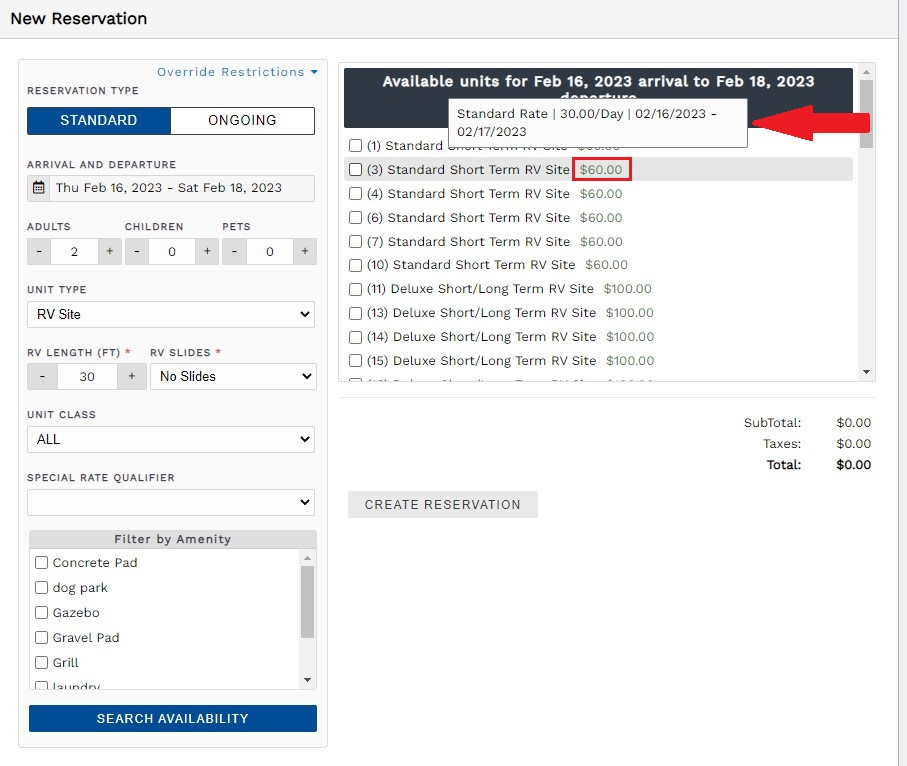Guest Portal

# Rate Calculation Descriptions

Any time a rate is calculated for a reservation, you might wonder exactly how that rate was calculated and which rates were used in the calculation. The rate calculation description now provides you with an easy way to view how the rate was calculated.

Note: The rate calculation description will only be available for reservations created after Feb 22, 2022. The calculation description will also show for any reservation unit where the rate was recalculated after this date, such as changing stay dates. Overriding the calculated rate by updating the charge amount will remove the calculation description.

To view the rate calculation description while making an internal reservation, hover over the reservation total to the right of each unit in the unit list displayed. This will give you a breakdown of which rate is being used for the reservation.View rate calculation while making an internal reservation

To view the rate calculation description after you make a reservation, first navigate to a reservation and click on the Units tab. Click on the reservation total to view the rate calculation description:

The rate calculation description will include every rate that was used, how much the rate was, and which dates the rate was applied. If you have multiple rates that apply during different time periods, it is possible that many different rates were used in the calculation. The description will clarify this to help you understand how the total was calculated.

Guests can also view the rate calculation description from the guest portal: